# Algebra Exit Tickets

Instructor: Nora Jarvis

Nora has a Master's degree in teaching, and has taught a variety of elementary grades.

Using exit tickets in your algebra class is a great way to check for understanding after a lesson. This lesson will get you started by providing some ideas for exit tickets that are specifically geared towards core Algebra concepts.

## Why use Exit Tickets?

At the end of a lesson, giving your students a short and simple problem to solve is a great way to make sure that they understood the material. As you collect your exit tickets, you can check for understanding. If some of your students seem to still be confused, you can reteach the concept or schedule time to check in with individual students.

When you assign exit tickets, plan for the students to have 2-3 minutes to complete the exit ticket. You want this to be short and quick so that you're not using up valuable class time.

## Solving Equations

Solving equations is an essential part of learning algebra. When creating an exit ticket, you'll want to consider what specific skill you are looking to assess. Do you want to make sure they can combine like terms? Then make sure that skill is needed to solve the equation. Do you want to make sure that students can solve an equation that requires multiple steps? If so, you'll need to make sure that your equation requires multiple steps.

Use these exit tickets to assess your students:

Solve this equation.

x-3 = 24

Solve this equation.

2x+4=30

Solve this equation.

x-10=40

## Linear Equations

Graphing linear equations is undoubtedly something that you will focus on in an algebra class. As you teach this concept, having students complete exit tickets is a helpful way of knowing what they understand and which areas they need additional support.

Use these exit tickets to assess your students:

Graph this linear equation.

y=2x+1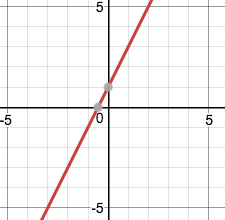Graph this linear equation.

y=6x-2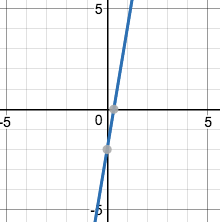Graph this linear equation.

4x=6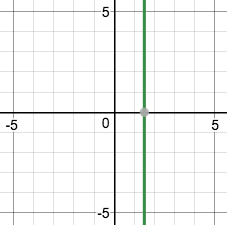As a major cornerstone of any algebra class, you'll want to assess your students' understanding of quadratic equations. Since this can be a lengthy process, you might start by having students work on smaller aspects of the process before they start solving equations.

Use these exit tickets to assess your students:

What is the standard form of the quadratic equation?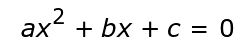Which are the known values of the quadratic equation?

• Answer: a, b, and c are all known values. You solve for x.

In the following equation, what are the values of a, b, and c?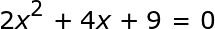• Answer: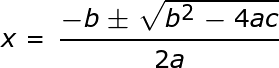To unlock this lesson you must be a Study.com Member.

### Register to view this lesson

Are you a student or a teacher?